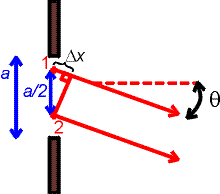Single-slit diffractionWhen we analyzed the two-slit experiment and the diffraction grating, we assumed that the individual slits were so narrow that they acted like point sources of waves. That is an accurate approximation if the slits are narrow compared to the wavelength. But in the case of wider slits, even a single slit causes a diffraction pattern, because every point in the slit serves as a source of a wave. Different parts of the slit have different distances to a given point on the screen, which causes interference effects.

Consider a slit of width a as shown. Consider two points of emission, 1 and 2, one from the upper half of the slit and one from the lower half, that are separated by a/2. For light that travels in the direction q, the contribution from point 1 will cancel the contribution from the point 2 if the difference D x is a half-integral number of wavelengths. Hence there will be a minimum in the intensity (dark spot) for those angles. Additional dark regions can found by dividing the slit into 4, 6, 8,...regions.

The general formula is

a sin q = m l

This formula looks just like the formula for the two-slit problem, but the interpretation is different in two ways:
(1) it describes minima (dark spots) rather than maxima; and
(2) m = 1,2,3,4,... and -1,-2,-3,-4,... but not m=0.

The single-slit diffraction pattern has a central maximum that covers the region between the m=1 dark spots. The first secondary maximum appears somewhere between the m=1 and m=2 minima (near but not exactly half way between them). The secondary maximum has a weaker intensity than the central maximum. The subsequent maxima are still weaker.

Interference and diffraction index      examples        Lecture index